# The Fundamentals of ggplot2 Explained

### February 26, 2014 | Alan

`ggplot2` is a data exploration and visualisation package written in R. Developed by Hadley Wickham, the principals of ggplot2 were defined in the Grammar of Graphics (Wilkinson, 2005) , which described the theoretical  division of graphs into semantic components. This approach of handling elements of a graph separately and building the features up in a series of layers allows for unmatched versatility and control.

### Learning Outcomes

This post covers the following:

• An explanation of the `qplot()` function.
• How to define a `ggplot()` object.
• An understanding to the concept of the aesthetics inside the `ggplot()` object.
• An understanding of layers and `geoms` objects.
• An understanding of applying `facets` , statistics, and `scales` to a plot.

We introduce these concepts as we work through an example.

### Data Source

In the following worked example, we are going to use  the dataset CO2, which comes pre-packaged with R. The CO2 uptake of six grass plants from Quebec and six grass plants from Mississippi was measured at several levels of ambient CO2 concentration. To assess the effects of temperature, half of the plants of each type were chilled overnight before the experiment was conducted. The dataset contains the following columns:

• `Plant`, which gives a unique identifier for each plant.
• `Type` is a factor, with levels Quebec and Mississippi giving the origin of the plant.
• `Treatment` is a factor, with levels non-chilled and chilled.
• `conc` is a numeric vector of ambient carbon dioxide concentrations (mL/L).
• `uptake` is a numeric vector of carbon dioxide uptake rates (umol/m2 sec).

Note that, for convenience, when we refer to “Quebec” and “Mississippi”, we are referring to the results for the plants in those cities, not the cities themselves!

In the `ggplot2` library, there are two types of plot that can be produced:

• `qplot()`
• `ggplot()`

We look at both in this blog post.

## Workflow

### Setting up the environment

Load the relevant library and dataset. Check that column names align with the Data Source description:

``````library(ggplot2)
library(plyr)
data(CO2)
names(CO2)
``````
``````##  "Plant"     "Type"      "Treatment" "conc"      "uptake"
``````

### Introducing the qplot function

`qplot()`, an abbreviation of “quick plot”, is a function belonging to the `ggplot2` library that is used for producing simple plots. `qplot()` is similar to the plot function in the base package for R, but has slightly more functionality.` qplot()` accepts `x` and `y` arguments, but also accepts “data”, where “data”“ is the data frame that houses the `x` and `y` coordinates. It is good practice to include the data argument, for example:

``````qplot(x, y, data = )
``````

Use `qplot()` to plot the uptake of CO2 against concentration level for plants across all data:

``````qplot(CO2\$conc, CO2\$uptake, data = CO2)
``````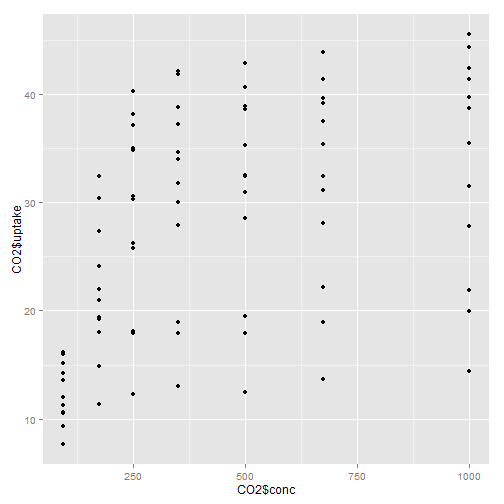As we can see, there is a large spread of data for each concentration level but we can split data further inside the `qplot()` function. To do this we use the shape and colour arguments to split data by `Treatment` and `Plant`:

``````qplot(CO2\$conc, CO2\$uptake, data = CO2, shape = CO2\$Treatment, colour = CO2\$Plant)
``````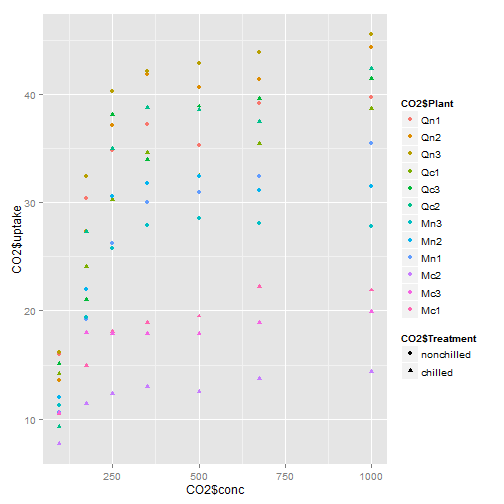Notice the difference in shape of the coloured points that identify Treatment. The default style of `qplot()` is a dot plot but by defining the `geom` attribute of a `qplot()` we can change the style. Applying “point” and “line” to `geom` gives:

``````qplot(CO2\$conc, CO2\$uptake, data = CO2, shape = CO2\$Treatment, colour = CO2\$Plant,
geom = c("point", "line"))
``````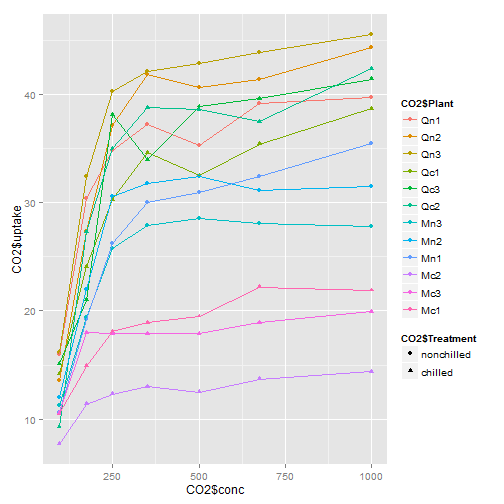Let’s also look at regional differences (`Type`) for CO2 uptake. To do this we use the `facets` argument to compare Quebec with Mississippi:

``````qplot(CO2\$conc, CO2\$uptake, data = transform(CO2, fct = CO2\$Type), shape = CO2\$Treatment,
colour = CO2\$Plant, geom = c("point", "line"), facets = ~fct)
``````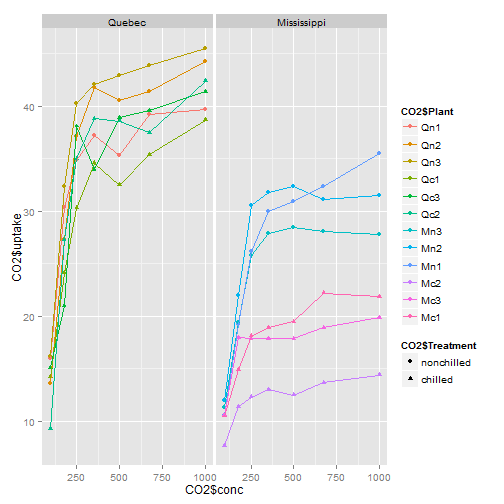Based on this plot, we can deduce the following:

• CO2 uptake looks like it has a logarithmic relationship when plotted against increasing concentration levels.
• Quebec has a higher CO2 uptake than Mississippi across all concentration levels.
• The variance in CO2 uptake is greater in Mississippi compared to Quebec.

You can find a complete list of arguments for the `qplot()` function using the following syntax:

``````?qplot
``````

which gives:

``````qplot(x, y, data=, color=, shape=, fill=, size=, alpha=, geom=, method=, formula=, facets=, xlim=, ylim= xlab=, ylab=, main=, sub=)
``````

where:

• `x` is a vector of x coordinates.
• `y` is a vector of y coordinates.
• `data` is the data frame housing `x` and `y`.
• `colour`, `shape`, `size` and `fill` are used to split data by features – legends are drawn automatically when any of these arguments are called.
• `alpha` is used to define the transparency of overlapping elements – ranges from 0 (clear) to 1 (opaque).
• `geom` is used to define the type to plot.
• `method` and `formula` are used to define regression lines and the model to use.
• `facets` splits the data into multiple graphs on one page.
• `xlim` and `ylim` are used to define the x and y axis limits.
• `xlab`, `ylab`, `main` and `sub` are used for labelling purposes – the x axis, y axis, title, and subtitle respectively.

Note that if you get into the habit of using the data argument, you can save yourself some time typing since you no longer need to prefix variables with their source dataframe . The last plot can also be generated with this code

`` qplot(conc, uptake, data= CO2, shape = Treatment, colour = Plant, geom = c("point", "line"), facets = ~Type) ``

### Introducing the ggplot function

The “gg” in `ggplot()` is an abbreviation of The Grammar of Graphics, a book by Leland Wilkinson, the principals of which `ggplot2` is derived from. The Grammar of Graphics describes the method of breaking graphs into elements and building up each element in a series of layers to control visual representation.

ggplot2: elegant graphics for data analysis by Hadley Wickham summarises the Grammar of Graphics nicely:

“In brief, the grammar tells us that a statistical graphic is a mapping from data to aesthetic attributes (colour, shape, size) of geometric objects (points, lines, bars). The plot may also contain statistical transformations of the data and is drawn on a specific coordinates system.”

A `ggplot` is made up of two main components – a `ggplot()` object and at least one `geom` layer.

#### The ggplot() object

The `ggplot()` object acts as a storage facility for the data. It is here where we define the data frame that houses the `x` and `y` coordinate values themselves and instructions on how to split the data. There are three ways to initialise a `ggplot()` object:

``````p <- ggplot()
p <- ggplot(data_frame)
p <- ggplot(data_frame, aes(x, y, ))
``````

Displaying the object `p` generated in the code chunk above would result in `Error: No layers in plot`. This is because you always need at least one layer for a `ggplot`.

#### Mapping aesthetics to data

The `aes()` aesthetic mapping function lives inside a `ggplot` object and is where we specify the set of plot attributes that remain constant throughout the subsequent layers (unless overwritten – more on this later).

We can consider the relationship between the `aes()` and `geoms` components as follows:

• The `aes()` function is the “how” – how data is stored, how data is split
• `geoms` is the “what”—what the data looks like. These are geometrical objects stored in subsequent layers.

#### Layers

We use the + operator to construct. By appending layers we can connect the “how” (aesthetics) to the “what” (geometric objects). Adding geometric, scale, facet and statistic layers to a `ggplot()` object is how to control virtually every visual aspect of the plot from the data contained in the object.

#### Adding a geometric object layer

A geometric object is used to define the style of the plot. Common geometric objects include:

• `geom_point()` which is used to draw a dot plot
• `geom_line()` used to draw a line plot
• `geom_bar()` used to draw a bar chart.

A single plot can have numerous `geom` layers, and it is also possible to overlay results from multiple data frames in one plot. Overwriting the aesthetic mapping that was defined in the `ggplot()` object can be done inside a `geom` object function (more on this later!).

Let’s apply the material covered so far for `ggplot()` to a worked example. To start this we:

1. create a `ggplot()` object
2. define the data frame and `x` and `y` coordinate values
3. add a single layer.

Notice that we don’t need the whole `title data_frame_name\$column_name` for our `x` and `y` values, because we are already directing to the CO2 data frame in the object definition:

``````p <- ggplot(CO2, aes(conc, uptake)) + geom_point()
p
``````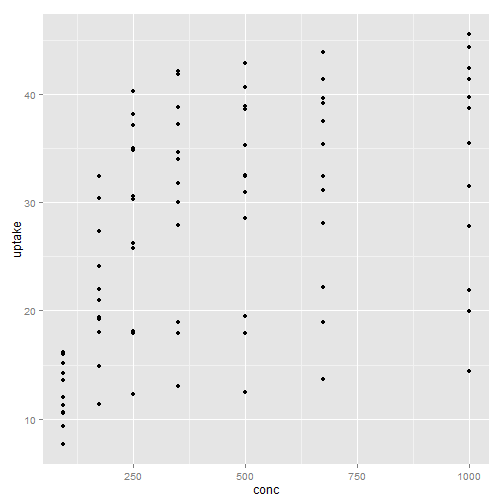Now, let’s investigate changing the aesthetic map attributes and the effect this has on the appearance of a plot:

1. define the data frame of interest
2. set `x` and `y` coordinate values inside the `aes()` function along with colouring by `Type`:
``````p <- ggplot(CO2, aes(x = conc, y = log(uptake), colour = Type)) + geom_point()
p
``````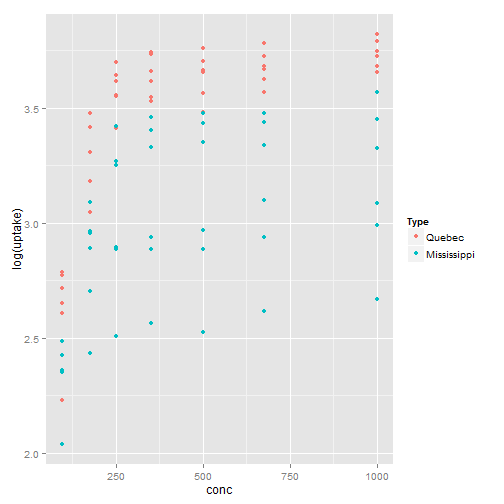As we can see, the data has now been split by column `Type`, and a colour-coded legend has automatically been generated. Data can be further split by using the `shape` and `fill` arguments. Offline, you should explore the different outputs from changing attributes of the `aes()` function and how this changes the content which is displayed.

#### Facets

Appending a facet layer to a `ggplot` generates the same plot for different subsets of data. Let’s reproduce the last plot in the `qplot` example using `ggplot`.  To do this we:

1. Define the `ggplot` object,
2. insert the data frame,
3. set the `x` and `y` coordinate values in the `aes()` function,
4. define the split by columns `Plant` and `Treatment`.
5. Append layers, `geom_point()` and `geom_line()` to mirror the previous plot. Add a `facet_grid()` to compare subsets of Type
``````p <- ggplot(CO2, aes(conc, uptake, colour = Plant, shape = Treatment)) + geom_point() +
geom_line()``````

Now we have our structure we can append a facet, add a `facet_grid()` to compare subsets of Type:

````q <- p ````+ facet_grid(~Type)
q``````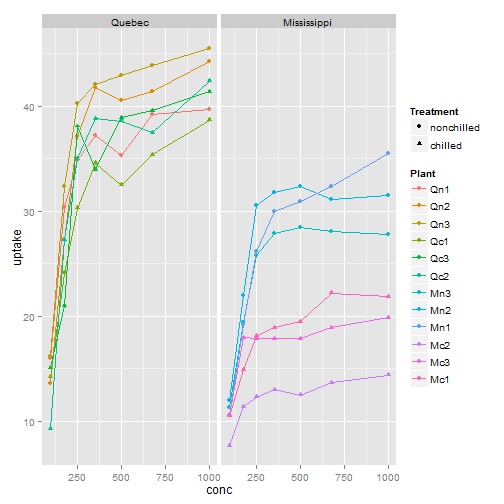The advantage of `ggplot()` over `qplot()` is its extreme flexibility – `ggplot()` was designed to handle much more complexity. The “shape” identifier for `Treatment` in the previous plot requires very good eyesight! Use `facet_grid()` to compare data further, let’s look at a `facet` arguments by columns `Treatment` and `Type`:

``````r <- p + facet_grid(Type ~ Treatment)
r``````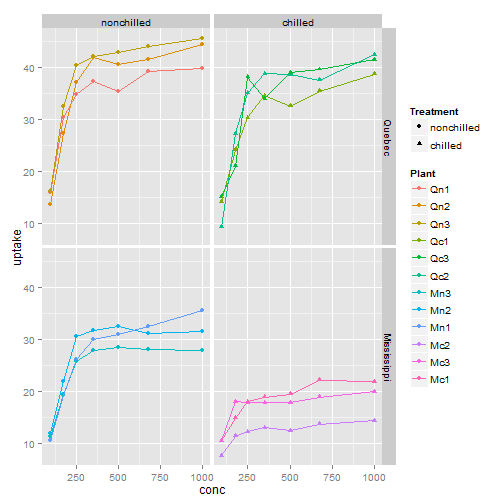From this plot we can deduce that for each region, overall, non-chilled plants saw a higher CO2 uptake than chilled. Now let us strengthen our deductions of the data by looking at some cold hard facts – statistics time!

#### Statistics

Exploratory data analysis can be done using the base packages in R, the results of which can be added to a `ggplot()` in the guise of a `geom` layer. Let’s look at the relationship between `concentration` of CO2 and `uptake` of CO2. To do this we remove the aesthetic split by `Plant` and the `geom_line()` layer and use `stat_summary()` to compute the mean of each concentration level:

``````p <- ggplot(CO2, aes(conc, uptake)) + geom_point() + facet_grid(Treatment ~
Type) + stat_summary(fun.y = mean, colour = "red", geom = "line")
p
``````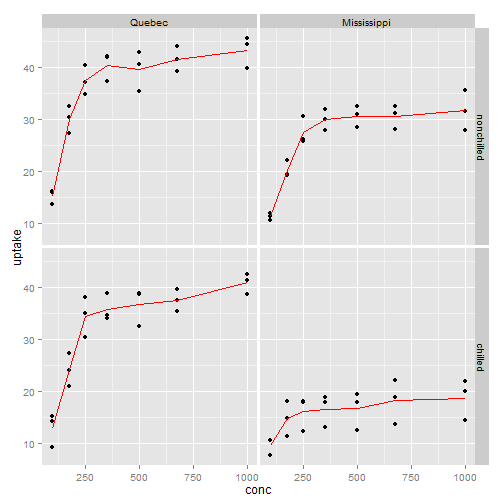Let’s annotate this plot with the mean values we have overlaid onto the plot. To do this we must deduce what these mean values are. Use the `ddply()` function in the `plyr` package to get a new data frame of the mean values of each group. To reflect how the data is split in the plot, let’s group our data by `Type`, `Treatment`, and `conc`:

``````# group_means <- ddply(CO2, c('Type','Treatment', 'conc'),
# function(hi)mean(hi\$uptake))
group_means <- ddply(CO2, .(Type, Treatment, conc), summarise, means = mean(uptake))
head(group_means, 10)
``````
``````##      Type  Treatment conc means
## 1  Quebec nonchilled   95 15.27
## 2  Quebec nonchilled  175 30.03
## 3  Quebec nonchilled  250 37.40
## 4  Quebec nonchilled  350 40.37
## 5  Quebec nonchilled  500 39.60
## 6  Quebec nonchilled  675 41.50
## 7  Quebec nonchilled 1000 43.17
## 8  Quebec    chilled   95 12.87
## 9  Quebec    chilled  175 24.13
## 10 Quebec    chilled  250 34.47
``````

The `means` column is the resulting mean of each group. Now use `geom_text()` to annotate the `group_means\$means` onto our plot. As mentioned above, multiple data frames can be overlaid onto one plot. For this new layer, set a new aesthetic mapping inside the `geom_text()` object. The text `label` along with `x` and `y` coordinates must be set. The syntax for this is as follows:

``````p <- ggplot(CO2, aes(conc, uptake)) + geom_point() + facet_grid(Treatment ~
Type) + stat_summary(fun.y = mean, colour = "red", geom = c("line", "point")) +
geom_text(data = group_means, aes(x = conc + 50, y = means - 4, label = round(means,
0)), colour = "red", inherit.aes = FALSE, parse = FALSE)
p
``````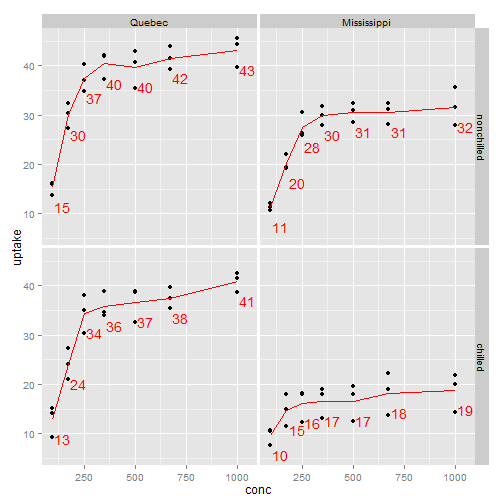#### Scales

Scales are used in `ggplot()` to administer control on the axes. `scale` covers everything from setting limits, through defining labels, to setting the granularity of the breaks in the data. For example, when setting limits, use:

• `scale_x_continuous()` to change the x axis limits
• `scale_y_continuous()` to change the y axis limits

The following code shows how to set y axis limits:

``````q <- p + scale_y_continuous(limits = c(0, 75))
q
``````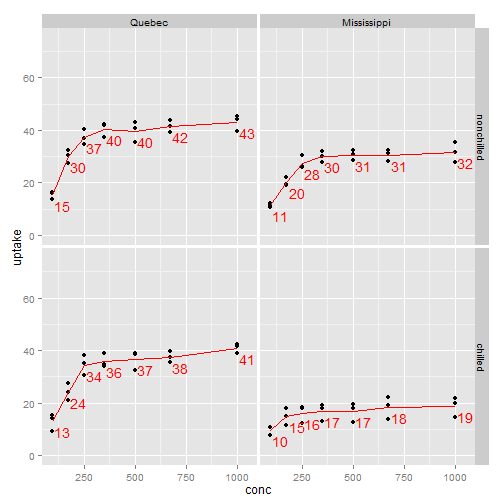Change the number of data points on the x axis:

``````r <- q + scale_x_continuous(breaks = seq(0, 1000, by = 100))
r
``````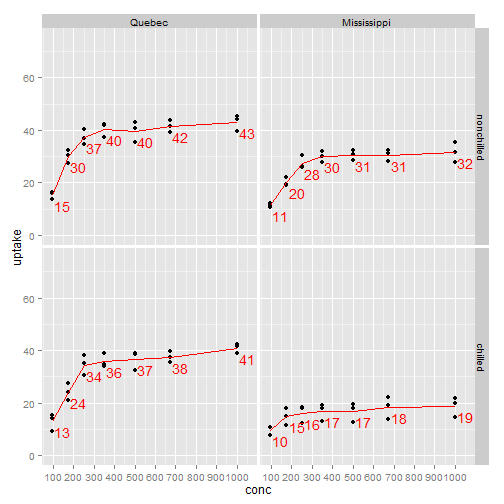We can now make observations about the average behaviour for each concentration level across region and treatment condition:

• On average, plants in Quebec have a higher CO2 uptake than those in Mississippi for both chilled and non-chilled conditions.
• On average, plants under non-chilled conditions have a higher CO2 uptake than chilled for both regions.
• On average, plants in Quebec under non-chilled conditions have the highest CO2 uptake across all concentration levels.
• On average, plants in Mississippi under chilled conditions have the lowest CO2 uptake across all concentration levels.

## What’s Next?

You can find more about ggplot in the following posts:

### Comments (7)

1.###### Robert Reply

February 21, 2015 at 11:15 pm

This was a great piece !

I found it very useful. I’m learning R for the first time.

I’m one of those learners that need to understand the essence (and logic behind) something in order to retain it …and avoid having to refer to a reference all the time.

Your expose was practical and succinct. Very useful.

Really appreciate it !

1.###### Annie O'Donnell Reply

February 27, 2015 at 10:27 am

You are very welcome Robert! Always happy to help and contribute to the community, if you have any questions feel free to ask away – annie.odonnell@aridhia.com

2.###### TAN THIAM HUATReply

May 28, 2017 at 3:42 am

Indeed, you give very clear explanations and examples, thank you.

1.###### Pamela Brankin Reply

June 12, 2017 at 2:41 pm

Thank you – please let us know if there are any other specific R packages that you might like to see a demonstration of!

3.###### Ranbir Reply

October 29, 2017 at 4:11 am

Hi, I am begineer, Request clarity in the following issue in using qplot function as described in your blog.

Rather than using

qplot(CO2\$conc, CO2\$uptake, data = transform(CO2, fct = CO2\$Type), shape = CO2\$Treatment,colour = CO2\$Plant, geom = c(“point”, “line”), facets = ~fct)

if i use

qplot(CO2\$conc, CO2\$uptake, data = CO2, shape = CO2\$Treatment,colour = CO2\$Plant, geom = c(“point”, “line”), facets = CO2\$Type)

I get error as below

Error in formula.default(eval(parse(text = x, keep.source = FALSE)[[1L]])) :
invalid formula

1.###### Harry Peaker Reply

October 30, 2017 at 12:09 pm

Hi Ranbir,

The `facets` argument of the qplot function needs to be a formula object. The easiest way to do this is with the ~ operator in the same way as the examples in the blog post.

So this modified version of your example will work.

`qplot(CO2\$conc, CO2\$uptake, data = CO2, shape = CO2\$Treatment,colour = CO2\$Plant, geom = c("point", "line"), facets = ~CO2\$Type)`

This site uses Akismet to reduce spam. Learn how your comment data is processed.